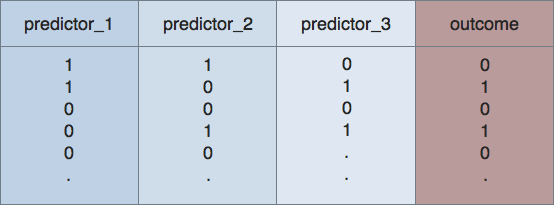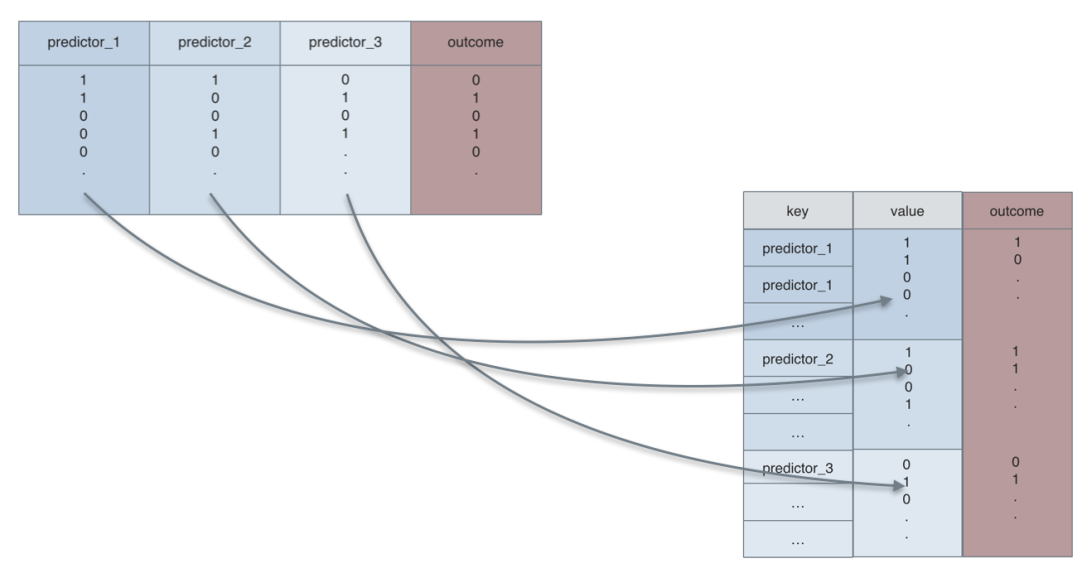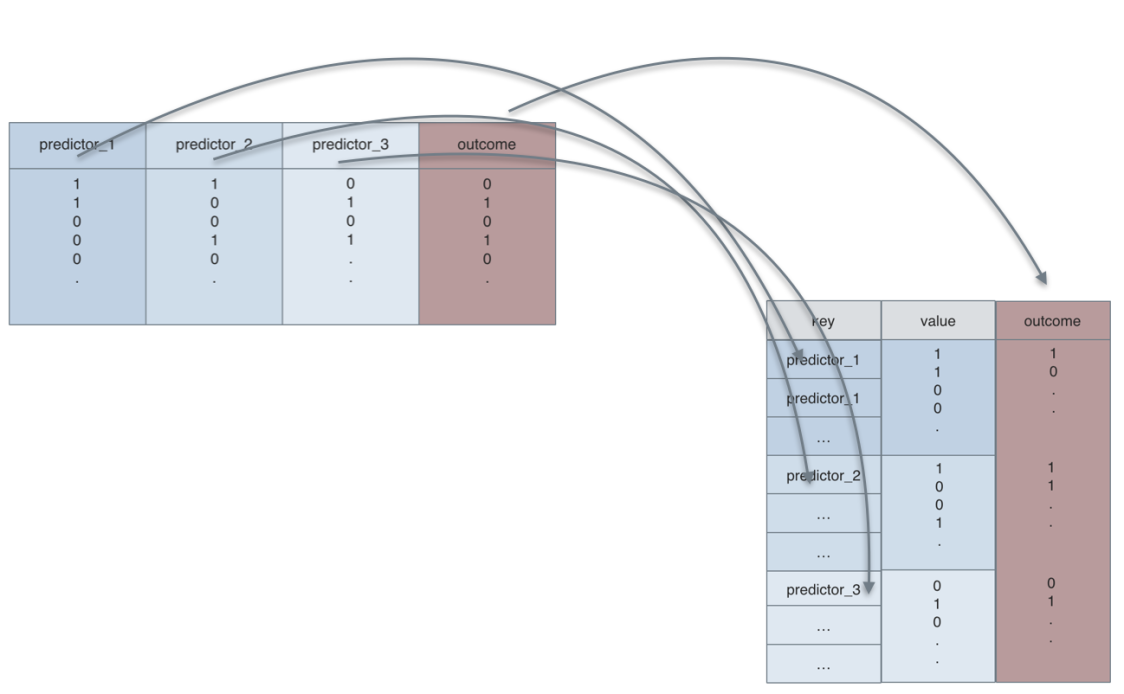# Long vs. wide format, and gather()

## July 04, 2016

Reading time ~3 minutes

reading time: 10 min.

A quite common task in data analysis is to change a dataset from wide to long format.

For example, this is a dataset in wide format:Is is called wide, as, well, it is wide – several columns side by side.

For example, assume, we have measured a number of predictors (here: predictor_1, predictor_2, predictor_3), and an outcome measure (here: outcome). In this case, each variable is dichotomous (either yes or no).

For plotting, eg. using ggplot2, we have to convert this format to long format. Long datasets look like this:So, non really surprising, the dataset is now longer than in wide format. Hence the name…

It was converted in a certain way. Look at the two figures (and the color scheme). The 4 columns are now 3: All of the predictors are now paired in two colums: key and value. In the key column we find the names of the former columns as entries; in the value column we find the entries of those columns as entries (check the colors).

We easily see that the variable outcome was not paired. Before and after the convertion, it happily resides in its own, whole column. We often want a non-paired column, as we need it for eg., facetting.

Let’s visualize this transformation. First, we look at the transformation of the values:Second, let’s look at the transformation of the keys (column headers), and the outcome variable, which will not be paires (remains in its own, complete, tidy column):So let’s see the tidyr and dplyr code:

library(dplyr)
library(tidyr)
library(ggplot2)

data(Wage, package = "ISLR")

Wage %>%
mutate(wage_f = ntile(wage, 2)) %>%  # bin it
mutate(wage_f = factor(wage_f, labels = c("low_wage","high_wage"))) %>%
select(health, race, wage_f) %>%
gather(key = predictor, value = pred_value, -wage_f) %>%
ggplot(aes(x = pred_value, fill = predictor)) + geom_bar() + facet_wrap(~wage_f) +
coord_flip()

## Warning: attributes are not identical across measure variables; they will
## be droppedThe plot itself is not particular useful as it is, but at times you will want such a type of plot. And many R functions built on the long format.

### New bar stacking with ggplot 2.2.0

Recently, ggplot2 2.2.0 was released. Among other news, stacking bar plot was improved. Here is a short demonstration.Load libraries`...… Continue reading

#### Crashkurs zur Erstellung von Barplots für Umfrage-Daten

Published on November 13, 2016

#### Some thoughts (and simulation) on overfitting

Published on November 12, 2016